# 12.3: Unit Cells and Basic Structures

Learning Objectives

Make sure you thoroughly understand the following essential ideas:

• The difference between square and hexagonal packing in two dimensions.
• The definition and significance of the unit cell.
• Sketch the three Bravais lattices of the cubic system, and calculate the number of atoms contained in each of these unit cells.
• Show how alternative ways of stacking three close-packed layers can lead to the hexagonal or cubic close packed structures.
• Explain the origin and significance of octahedral and tetrahedral holes in stacked close-packed layers, and show how they can arise.

## Close-Packing of Identical Spheres

Crystals are of course three-dimensional objects, but we will begin by exploring the properties of arrays in two-dimensional space. This will make it easier to develop some of the basic ideas without the added complication of getting you to visualize in 3-D — something that often requires a bit of practice. Suppose you have a dozen or so marbles. How can you arrange them in a single compact layer on a table top? Obviously, they must be in contact with each other in order to minimize the area they cover. It turns out that there are two efficient ways of achieving this:The essential difference here is that any marble within the interior of the square-packed array is in contact with four other marbles, while this number rises to six in the hexagonal-packed arrangement. It should also be apparent that the latter scheme covers a smaller area (contains less empty space) and is therefore a more efficient packing arrangement. If you are good at geometry, you can show that square packing covers 78 percent of the area, while hexagonal packing yields 91 percent coverage.

If we go from the world of marbles to that of atoms, which kind of packing would the atoms of a given element prefer?If the atoms are identical and are bound together mainly by dispersion forces which are completely non-directional, they will favor a structure in which as many atoms can be in direct contact as possible. This will, of course, be the hexagonal arrangement.

Directed chemical bonds between atoms have a major effect on the packing. The version of hexagonal packing shown at the right occurs in the form of carbon known as graphite which forms 2-dimensional sheets. Each carbon atom within a sheet is bonded to three other carbon atoms. The result is just the basic hexagonal structure with some atoms missing.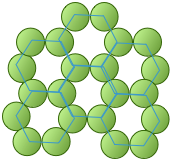The coordination number of 3 reflects the sp2-hybridization of carbon in graphite, resulting in plane-trigonal bonding and thus the sheet structure. Adjacent sheets are bound by weak dispersion forces, allowing the sheets to slip over one another and giving rise to the lubricating and flaking properties of graphite.

## Lattices

The underlying order of a crystalline solid can be represented by an array of regularly spaced points that indicate the locations of the crystal's basic structural units. This array is called a crystal lattice. Crystal lattices can be thought of as being built up from repeating units containing just a few atoms. These repeating units act much as a rubber stamp: press it on the paper, move ("translate") it by an amount equal to the lattice spacing, and stamp the paper again.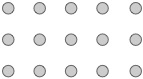The gray circles represent a square array of lattice points.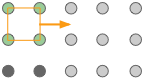The orange square is the simplest unit cell that can be used to define the 2-dimensional lattice.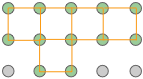Building out the lattice by moving ("translating") the unit cell in a series of steps,

Although real crystals do not actually grow in this manner, this process is conceptually important because it allows us to classify a lattice type in terms of the simple repeating unit that is used to "build" it. We call this shape the unit cell. Any number of primitive shapes can be used to define the unit cell of a given crystal lattice. The one that is actually used is largely a matter of convenience, and it may contain a lattice point in its center, as you see in two of the unit cells shown here. In general, the best unit cell is the simplest one that is capable of building out the lattice.Shown above are unit cells for the close-packed square and hexagonal lattices we discussed near the start of this lesson. Although we could use a hexagon for the second of these lattices, the rhombus is preferred because it is simpler.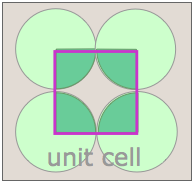Notice that in both of these lattices, the corners of the unit cells are centered on a lattice point. This means that an atom or molecule located on this point in a real crystal lattice is shared with its neighboring cells. As is shown more clearly here for a two-dimensional square-packed lattice, a single unit cell can claim "ownership" of only one-quarter of each molecule, and thus "contains" 4 × ¼ = 1 molecule.The unit cell of the graphite form of carbon is also a rhombus, in keeping with the hexagonal symmetry of this arrangement. Notice that to generate this structure from the unit cell, we need to shift the cell in both the x- and y- directions in order to leave empty spaces at the correct spots. We could alternatively use regular hexagons as the unit cells, but the x+y shifts would still be required, so the simpler rhombus is usually preferred. As you will see in the next section, the empty spaces within these unit cells play an important role when we move from two- to three-dimensional lattices.

## Cubic crystals

In order to keep this lesson within reasonable bounds, we are limiting it mostly to crystals belonging to the so-called cubic system. In doing so, we can develop the major concepts that are useful for understanding more complicated structures (as if there are not enough complications in cubics alone!) But in addition, it happens that cubic crystals are very commonly encountered; most metallic elements have cubic structures, and so does ordinary salt, sodium chloride.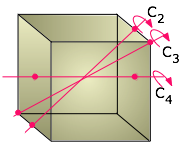We usually think of a cubic shape in terms of the equality of its edge lengths and the 90° angles between its sides, but there is another way of classifying shapes that chemists find very useful. This is to look at what geometric transformations (such as rotations around an axis) we can perform that leave the appearance unchanged. For example, you can rotate a cube 90° around an axis perpendicular to any pair of its six faces without making any apparent change to it. We say that the cube possesses three mutually perpendicular four-fold rotational axes, abbreviated C4 axes. But if you think about it, a cube can also be rotated around the axes that extend between opposite corners; in this case, it takes three 120° rotations to go through a complete circle, so these axes (also four in number) are three-fold or C3 axes.

Cubic crystals belong to one of the seven crystal systems whose lattice points can be extended indefinitely to fill three-dimensional space and which can be constructed by successive translations (movements) of a primitive unit cell in three dimensions. As we will see below, the cubic system, as well as some of the others, can have variants in which additional lattice points can be placed at the center of the unit or at the center of each face.

### The three types of cubic lattices

The three Bravais lattices which form the cubic crystal system are shown here.Structural examples of all three are known, with body- and face-centered (BCC and FCC) being much more common; most metallic elements crystallize in one of these latter forms. But although the simple cubic structure is uncommon by itself, it turns out that many BCC and FCC structures composed of ions can be regarded as interpenetrating combinations of two simple cubic lattices, one made up of positive ions and the other of negative ions. Notice that only the FCC structure, which we will describe below, is a close-packed lattice within the cubic system.

## Close-packed lattices in three dimensions

Close-packed lattices allow the maximum amount of interaction between atoms. If these interactions are mainly attractive, then close-packing usually leads to more energetically stable structures. These lattice geometries are widely seen in metallic, atomic, and simple ionic crystals.

As we pointed out above, hexagonal packing of a single layer is more efficient than square-packing, so this is where we begin. Imagine that we start with the single layer of green atoms shown below. We will call this the A layer. If we place a second layer of atoms (orange) on top of the A-layer, we would expect the atoms of the new layer to nestle in the hollows in the first layer. But if all the atoms are identical, only some of these void spaces will be accessible.In the diagram on the left, notice that there are two classes of void spaces between the A atoms; one set (colored blue) has a vertex pointing up, while the other set (not colored) has down-pointing vertices. Each void space constitutes a depression in which atoms of a second layer (the B-layer) can nest. The two sets of void spaces are completely equivalent, but only one of these sets can be occupied by a second layer of atoms whose size is similar to those in the bottom layer. In the illustration on the right above we have arbitrarily placed the B-layer atoms in the blue voids, but could just as well have selected the white ones.

### Two choices for the third layer lead to two different close-packed lattice types

Now consider what happens when we lay down a third layer of atoms. These will fit into the void spaces within the B-layer. As before, there are two sets of these positions, but unlike the case described above, they are not equivalent.The atoms in the third layer are represented by open blue circles in order to avoid obscuring the layers underneath. In the illustration on the left, this third layer is placed on the B-layer at locations that are directly above the atoms of the A-layer, so our third layer is just a another A layer. If we add still more layers, the vertical sequence A-B-A-B-A-B-A... repeats indefinitely.

In the diagram on the right above, the blue atoms have been placed above the white (unoccupied) void spaces in layer A. Because this third layer is displaced horizontally (in our view) from layer A, we will call it layer C. As we add more layers of atoms, the sequence of layers is A-B-C-A-B-C-A-B-C..., so we call it ABC packing.

For the purposes of clarity, only three atoms of the A and C layers are shown in these diagrams. But in reality, each layer consists of an extended hexagonal array; the two layers are simply displaced from one another.These two diagrams that show exploded views of the vertical stacking further illustrate the rather small fundamental difference between these two arrangements— but, as you will see below, they have widely divergent structural consequences. Note the opposite orientations of the A and C layers

### The Hexagonal closed-packed structure

The HCP stacking shown on the left just above takes us out of the cubic crystal system into the hexagonal system, so we will not say much more about it here except to point out each atom has 12 nearest neighbors: six in its own layer, and three in each layer above and below it.### The cubic close-packed structure

Below we reproduce the FCC structure that was shown above.You will notice that the B-layer atoms form a hexagon, but this is a cubic structure. How can this be? The answer is that the FCC stack is inclined with respect to the faces of the cube, and is in fact coincident with one of the three-fold axes that passes through opposite corners. It requires a bit of study to see the relationship, and we have provided two views to help you. The one on the left shows the cube in the normal isometric projection; the one on the right looks down upon the top of the cube at a slightly inclined angle.Both the CCP and HCP structures fill 74 percent of the available space when the atoms have the same size. You should see that the two shaded planes cutting along diagonals within the interior of the cube contain atoms of different colors, meaning that they belong to different layers of the CCP stack. Each plane contains three atoms from the B layer and three from the C layer, thus reducing the symmetry to C3, which a cubic lattice must have.

### The FCC unit cell

The figure below shows the the face-centered cubic unit cell of a cubic-close packed lattice.How many atoms are contained in a unit cell? Each corner atom is shared with eight adjacent unit cells and so a single unit cell can claim only 1/8 of each of the eight corner atoms. Similarly, each of the six atoms centered on a face is only half-owned by the cell. The grand total is then (8 × 1/8) + (6 × ½) = 4 atoms per unit cell.

## Interstitial Void Spaces

The atoms in each layer in these close-packing stacks sit in a depression in the layer below it. As we explained above, these void spaces are not completely filled. (It is geometrically impossible for more than two identical spheres to be in contact at a single point.) We will see later that these interstitial void spaces can sometimes accommodate additional (but generally smaller) atoms or ions.If we look down on top of two layers of close-packed spheres, we can pick out two classes of void spaces which we call tetrahedral and octahedral holes.

### Tetrahedral holes

If we direct our attention to a region in the above diagram where a single atom is in contact with the three atoms in the layers directly below it, the void space is known as a tetrahedral hole. A similar space will be be found between this single atom and the three atoms (not shown) that would lie on top of it in an extended lattice. Any interstitial atom that might occupy this site will interact with the four atoms surrounding it, so this is also called a four-coordinate interstitial space.Don't be misled by this name; the boundaries of the void space are spherical sections, not tetrahedra. The tetrahedron is just an imaginary construction whose four corners point to the centers of the four atoms that are in contact.

### Octahedral holes

Similarly, when two sets of three trigonally-oriented spheres are in close-packed contact, they will be oriented 60° apart and the centers of the spheres will define the six corners of an imaginary octahedron centered in the void space between the two layers, so we call these octahedral holes or six-coordinate interstitial sites. Octahedral sites are larger than tetrahedral sites.An octahedron has six corners and eight sides. We usually draw octahedra as a double square pyramid standing on one corner (left), but in order to visualize the octahedral shape in a close-packed lattice, it is better to think of the octahedron as lying on one of its faces (right).Each sphere in a close-packed lattice is associated with one octahedral site, whereas there are only half as many tetrahedral sites. This can be seen in this diagram that shows the central atom in the B layer in alignment with the hollows in the C and A layers above and below.The face-centered cubic unit cell contains a single octahedral hole within itself, but octahedral holes shared with adjacent cells exist at the centers of each edge. Each of these twelve edge-located sites is shared with four adjacent cells, and thus contributes (12 × ¼) = 3 atoms to the cell. Added to the single hole contained in the middle of the cell, this makes a total of 4 octahedral sites per unit cell. This is the same as the number we calculated above for the number of atoms in the cell.

## Common cubic close-packed structures

It can be shown from elementary trigonometry that an atom will fit exactly into an octahedral site if its radius is 0.414 as great as that of the host atoms. The corresponding figure for the smaller tetrahedral holes is 0.225.

Many pure metals and compounds form face-centered cubic (cubic close- packed) structures. The existence of tetrahedral and octahedral holes in these lattices presents an opportunity for "foreign" atoms to occupy some or all of these interstitial sites. In order to retain close-packing, the interstitial atoms must be small enough to fit into these holes without disrupting the host CCP lattice. When these atoms are too large, which is commonly the case in ionic compounds, the atoms in the interstitial sites will push the host atoms apart so that the face-centered cubic lattice is somewhat opened up and loses its close-packing character.

### The rock-salt structure

Alkali halides that crystallize with the "rock-salt" structure exemplified by sodium chloride can be regarded either as a FCC structure of one kind of ion in which the octahedral holes are occupied by ions of opposite charge, or as two interpenetrating FCC lattices made up of the two kinds of ions. The two shaded octahedra illustrate the identical coordination of the two kinds of ions; each atom or ion of a given kind is surrounded by six of the opposite kind, resulting in a coordination expressed as (6:6).How many NaCl units are contained in the unit cell? If we ignore the atoms that were placed outside the cell in order to construct the octahedra, you should be able to count fourteen "orange" atoms and thirteen "blue" ones. But many of these are shared with adjacent unit cells.

An atom at the corner of the cube is shared by eight adjacent cubes, and thus makes a 1/8 contribution to any one cell. Similarly, the center of an edge is common to four other cells, and an atom centered in a face is shared with two cells. Taking all this into consideration, you should be able to confirm the following tally showing that there are four AB units in a unit cell of this kind.

Orange Blue
8 at corners: 8 x 1/8 = 1 12 at edge centers: 12 x ¼ = 3
6 at face centers: 6 x ½ = 3 1 at body center = 1
total: 4 total: 4

If we take into consideration the actual sizes of the ions (Na+ = 116 pm, Cl = 167 pm), it is apparent that neither ion will fit into the octahedral holes with a CCP lattice composed of the other ion, so the actual structure of NaCl is somewhat expanded beyond the close-packed model.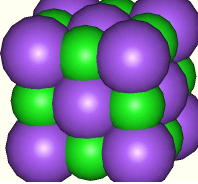The space-filling model on the right depicts a face-centered cubic unit cell of chloride ions (purple), with the sodium ions (green) occupying the octahedral sites.

### The zinc-blende structure: using some tetrahedral holes

Since there are two tetrahedral sites for every atom in a close-packed lattice, we can have binary compounds of 1:1 or 1:2 stoichiometry depending on whether half or all of the tetrahedral holes are occupied. Zinc-blende is the mineralogical name for zinc sulfide, ZnS. An impure form known as sphalerite is the major ore from which zinc is obtained.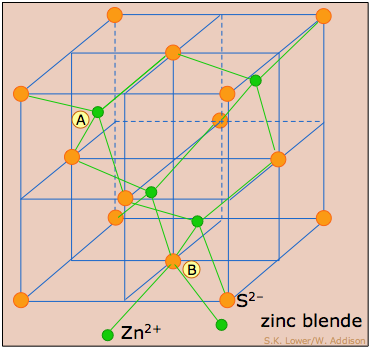This structure consists essentially of a FCC (CCP) lattice of sulfur atoms (orange) (equivalent to the lattice of chloride ions in NaCl) in which zinc ions (green) occupy half of the tetrahedral sites. As with any FCC lattice, there are four atoms of sulfur per unit cell, and the the four zinc atoms are totally contained in the unit cell. Each atom in this structure has four nearest neighbors, and is thus tetrahedrally coordinated.

It is interesting to note that if all the atoms are replaced with carbon, this would correspond to the diamond structure.

### The fluorite structure: all tetrahedral sites occupied

Fluorite, CaF2, having twice as many ions of fluoride as of calcium, makes use of all eight tetrahedral holes in the CPP lattice of calcium ions (orange) depicted here. To help you understand this structure, we have shown some of the octahedral sites in the next cell on the right; you can see that the calcium ion at A is surrounded by eight fluoride ions, and this is of course the case for all of the calcium sites. Since each fluoride ion has four nearest-neighbor calcium ions, the coordination in this structure is described as (8:4).Although the radii of the two ions (F= 117 pm, Ca2+ = 126 pm does not allow true close packing, they are similar enough that one could just as well describe the structure as a FCC lattice of fluoride ions with calcium ions in the octahedral holes.

## Simple- and body-centered cubic structures

In Section 4 we saw that the only cubic lattice that can allow close packing is the face-centered cubic structure. The simplest of the three cubic lattice types, the simple cubic lattice, lacks the hexagonally-arranged layers that are required for close packing. But as shown in this exploded view, the void space between the two square-packed layers of this cell constitutes an octahedral hole that can accommodate another atom, yielding a packing arrangement that in favorable cases can approximate true close-packing. Each second-layer B atom (blue) resides within the unit cell defined the A layers above and below it.The A and B atoms can be of the same kind or they can be different. If they are the same, we have a body-centered cubic lattice. If they are different, and especially if they are oppositely-charged ions (as in the CsCl structure), there are size restrictions: if the B atom is too large to fit into the interstitial space, or if it is so small that the A layers (which all carry the same electric charge) come into contact without sufficient A-B coulombic attractions, this structural arrangement may not be stable.

### The cesium chloride structure

CsCl is the common model for the BCC structure. As with so many other structures involving two different atoms or ions, we can regard the same basic structure in different ways. Thus if we look beyond a single unit cell, we see that CsCl can be represented as two interpenetrating simple cubic lattices in which each atom occupies an octahedral hole within the cubes of the other lattice.